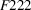International Tables for Crystallography (2006). Vol. A, ch. 15.3, pp. 900-903https://doi.org/10.1107/97809553602060000535

## Contents

• 15.3. Examples of the use of normalizers  (pp. 900-903)
• 15.3.1. Introduction  (p. 900) | html | pdf |
• 15.3.2. Equivalent point configurations, equivalent Wyckoff positions and equivalent descriptions of crystal structures  (pp. 900-901) | html | pdf |
• 15.3.3. Equivalent lists of structure factors  (pp. 901-902) | html | pdf |
• 15.3.4. Euclidean- and affine-equivalent sub- and supergroups  (pp. 902-903) | html | pdf |
• 15.3.5. Reduction of the parameter regions to be considered for geometrical studies of point configurations  (p. 903) | html | pdf |
• References | html | pdf |
• Tables
• Table 15.3.3.1. Changes of structure-factor phases for the equivalent descriptions of a crystal structure in(p. 902) | html | pdf |